## 介绍

### 关于 Kalman Filter

Kalman Filter 算法，是一种递推预测滤波算法，算法中即涉及到滤波，也涉及到对下一时刻数据的预测。Kalman Filter 由一系列递归数学公式描述。它们提供了一种高效可计算的方法来估计过程的状态，并使估计均方误差最小。卡尔曼滤波器应用广泛且功能强大：它可以估计信号的过去和当前状态，甚至能估计将来的状态，即使并不知道模型的确切性质。

Kalman Filter 也可以被认为是一种数据融合算法（Data fused algorithm），已有50多年的历史，是当今使用最重要和最常见的数据融合算法之一。Kalman Filter 的巨大成功归功于其小的计算需求，优雅的递归属性以及作为具有高斯误差统计的一维线性系统的最优估计器的状态。

Kalman Filter 只能减小均值为0的测量噪声带来的影响。只要噪声期望为0，那么不管方差多大，只要迭代次数足够多，那效果都很好。反之，噪声期望不为0，那么估计值就还是与实际值有偏差。

## 图形解释

### 对小车的位置进行估计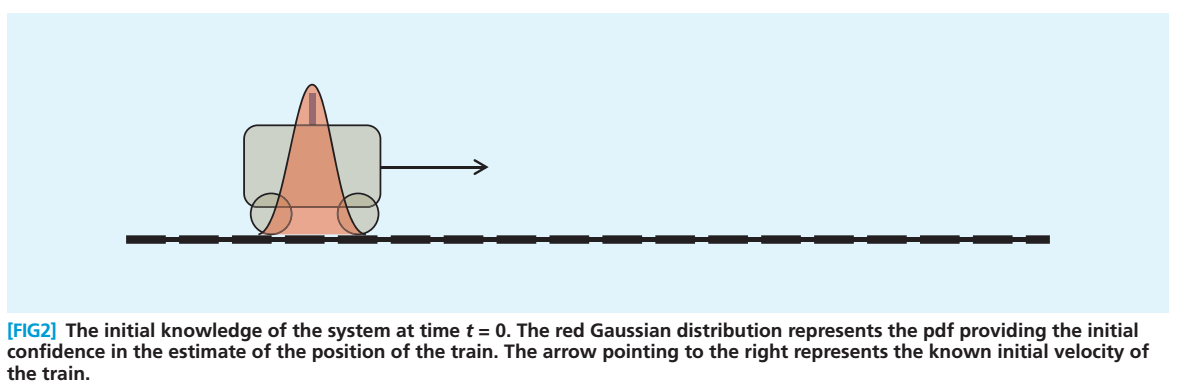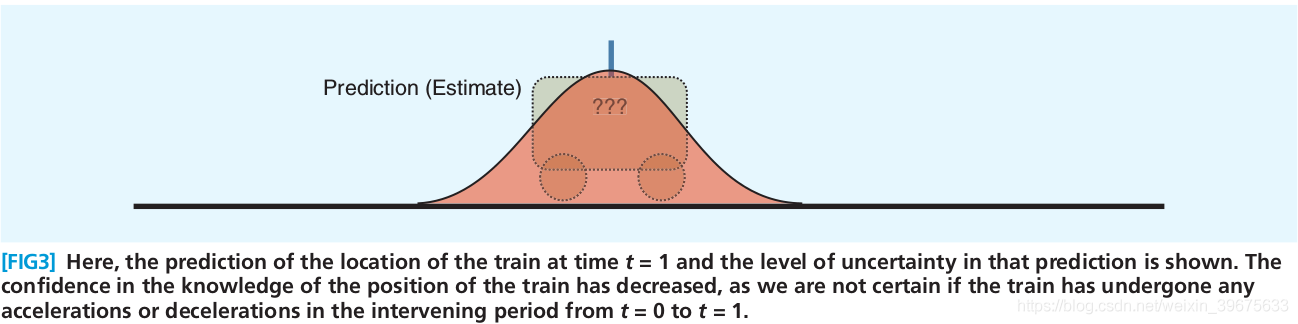### 估计中加入测量（measurement）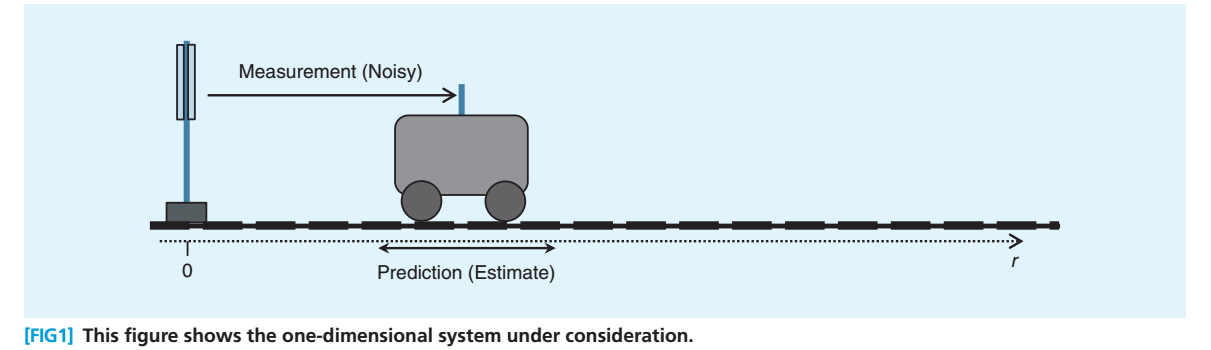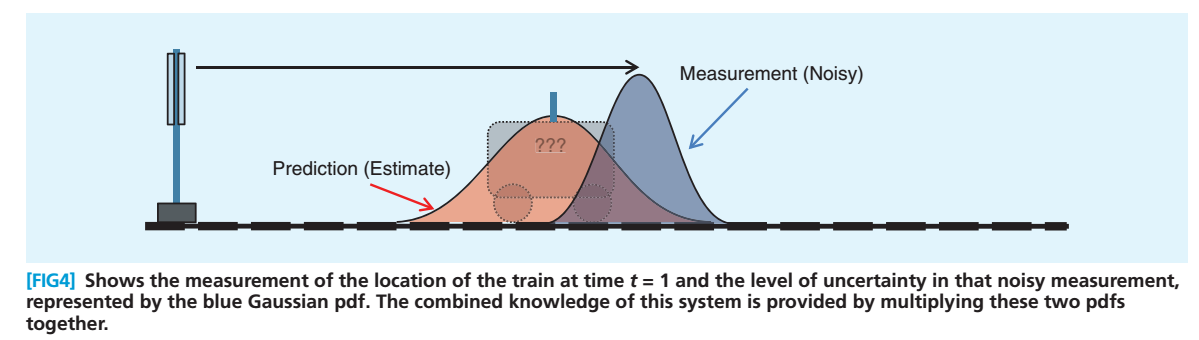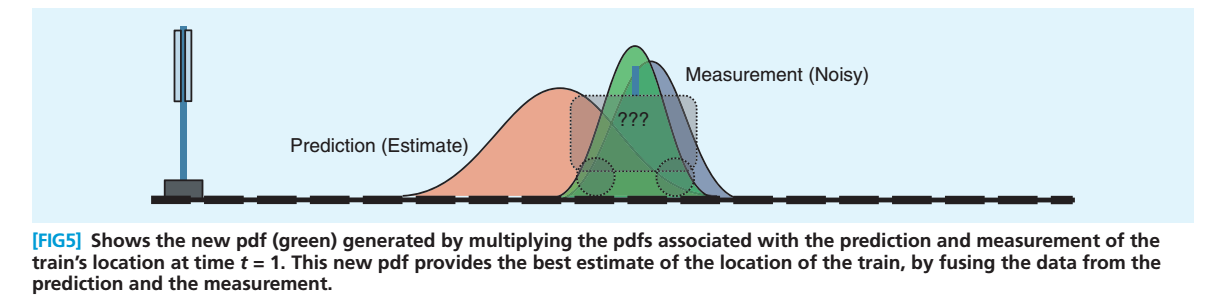## 离散卡尔曼滤波器

1960年，Kalman 发表了他著名的用递归方法解决离散数据线性滤波问题的文章。这部分内容主要来自。

### 被估计的过程状态

$x_k = F_k \cdot x_{k-1}+ B_k \cdot u_{k-1}+w_{k-1}$

$z_k=H_k \cdot x_k+v_k$

$\left\{\begin{matrix} p(w) \sim N(0,Q)\\ p(v) \sim N(0,R) \end{matrix}\right.$

### 离散卡尔曼滤波器算法

Kalman Filter 用反馈控制的方法估计过程状态：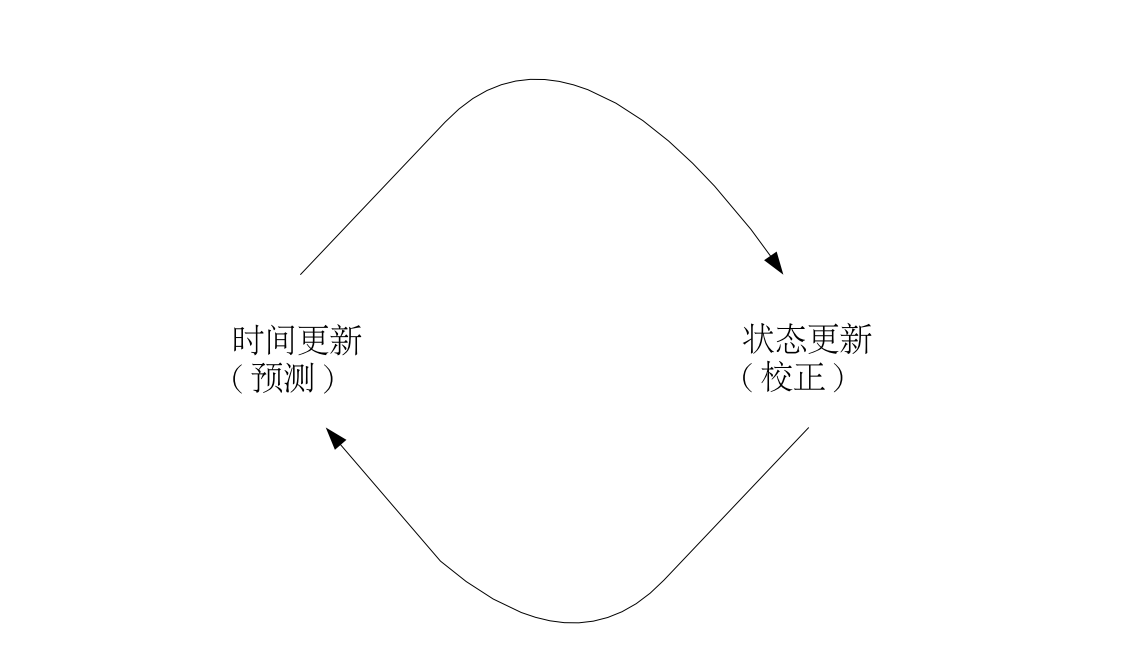#### 时间更新方程

$\hat{x_k}^-=F \cdot \hat{x_{k-1}}+B \cdot u_{k-1}$
$P_k^-=F \cdot P_{k-1} \cdot F^T + Q$

• $\hat{x_k}^- \in R^n$$-$ 代表先验，$\hat{}$ 代表估计），为在已知第 $k$ 步以前状态情况下，第 $k$ 步的先验状态估计。
• $\hat{x_k} \in R^n$ 为已知测量变量 $z_k$ 时，第 $k$ 步的后验状态估计。
• $F$ 为将上一时刻 $k-1$ 的状态线性映射到当前时刻 $k$ 的状态转换矩阵，实际中 $F$ 应随时间变化，这里假设为常数。
• $B$ 为应用于控制向量 $u_k$ 的控制输入矩阵，假设为常数。

$\left\{\begin{matrix} e_k^- \equiv x_k - \hat{x_k}^-\\ e_k \equiv x_k - \hat{x_k} \end{matrix}\right.$

$\left\{\begin{matrix} P_k^- = E[e_k^-{e_k^-}^T] \\ P_k = E[e_ke_k^T] \end{matrix}\right.$

$P_k^-=E[(x_k-x_k^-)(x_k-x_k^-)^T]$

$P_k^-=E[(F \cdot (x_{k-1}-\hat{x}_{k-1})+w)(F \cdot (x_{k-1}-\hat{x}_{k-1})+w )^T]$

$P_k^-=E[(F\cdot e_{k-1}+w)(F \cdot e_{k-1}+w)^T]$

$P_k^- = E[F\cdot e_{k-1} \cdot w^T+F\cdot e_{k-1} \cdot e_{k-1}^T \cdot F^T+w\cdot e_{k-1}^T\cdot F^T+ w\cdot w^T]$

$P_k^-=F \cdot P_{k-1} \cdot F^T + Q$

#### 测量（状态）更新方程

$\hat{x}_k=\hat{x}_k^-+K_k(z_k-H\cdot \hat{x}_k^-)$
$P_k=P_k^--K_k\cdot H\cdot P_k^-$
$K_k=P_k^-\cdot H^T(H\cdot P_k^-\cdot H^T+R)^{-1}$

$E[x_k]=\hat{x}_k$

$E[(x_k-\hat{x}_k)(x_k-\hat{x})^T]=P_k$

$p(x_k|z_k)\sim N(E[x_k],E[(x_k-\hat{x}_k)(x_k-\hat{x}_k)^T])$

$p(x_k|z_k)\sim N(\hat{x}_k, P_k)$

$K_k=P_k^-\cdot H^T(H\cdot P_k^-\cdot H^T+R)^{-1}$

$K_k = \frac{P_k^-\cdot H^T}{H\cdot P_k^-\cdot H^T+R}$

$\lim_{R\rightarrow 0}K_k=H^{-1}$

$\lim_{P_k^- \rightarrow 0} K_k = 0$

#### 迭代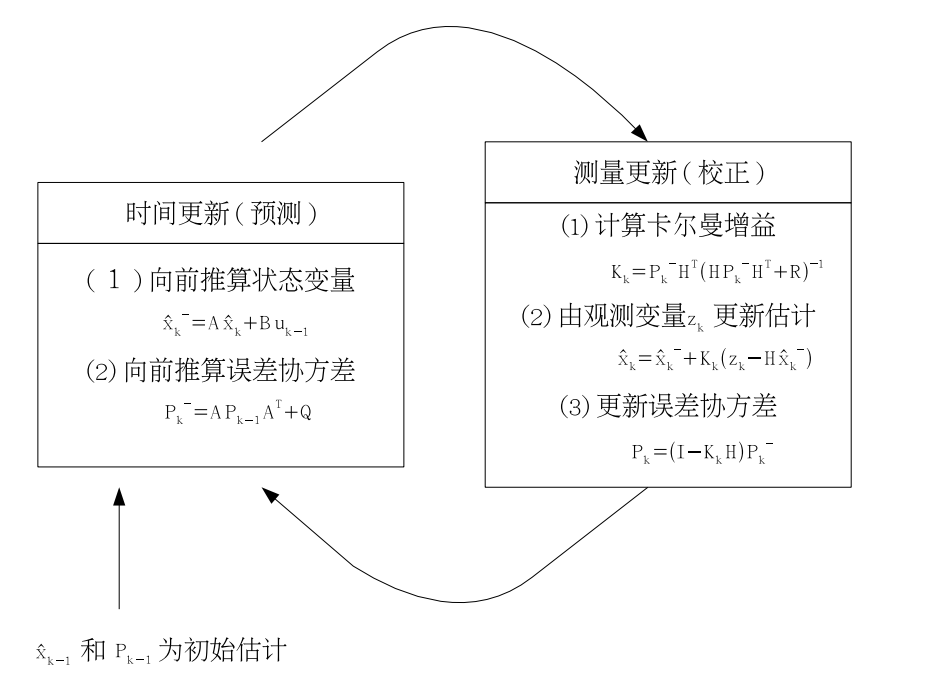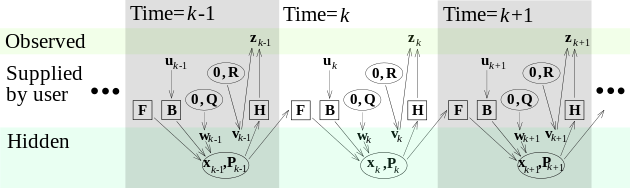## 实践：估计随机常数

### 状态模型

$x_k=x_{k-1}+w$

$z_k=x_k+v$

### 滤波器方程和参数

$\hat{x}_k^- = \hat{x}_{k-1}+w$

$P_k^-=P_{k-1}+Q$

$K_k=P_k^-(P_k^-+R)^{-1}=\frac{P_k^-}{P_k^-+R}$

$\hat{x}_k=\hat{x}_k^-+K_k(z_k-\hat{x}_k^-)$

$P_k=P_k^--K_k\cdot P_k^-$

## Reference

 R. Faragher, “Understanding the Basis of the Kalman Filter Via a Simple and Intuitive Derivation [Lecture Notes]”, IEEE Signal Processing Magazine, vol. 29, no. 5, pp. 128–132, Sep. 2012.

 G. Welch and G. Bishop, “An Introduction to the Kalman Filter”, p. 16, 2006.

 Fitzgerald, Robert J. “Divergence of the Kalman filter”, Automatic Control IEEE Transactions on 16.6(1971):736-747.

 如何通俗并尽可能详细解释卡尔曼滤波？ - 肖畅的回答 - 知乎 https://www.zhihu.com/question/23971601/answer/46480923